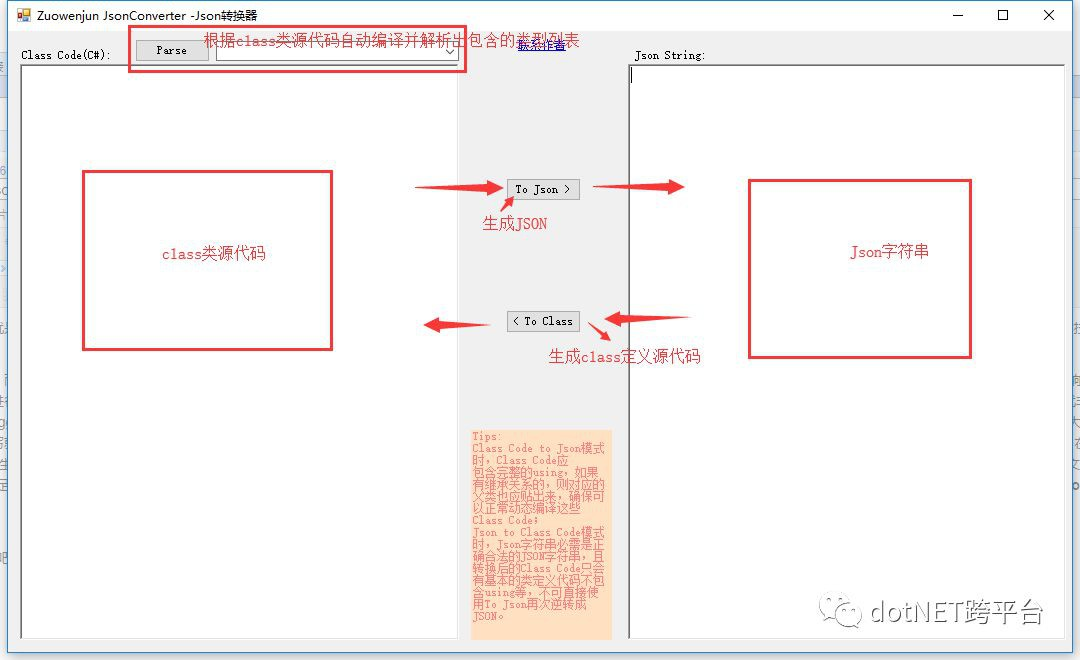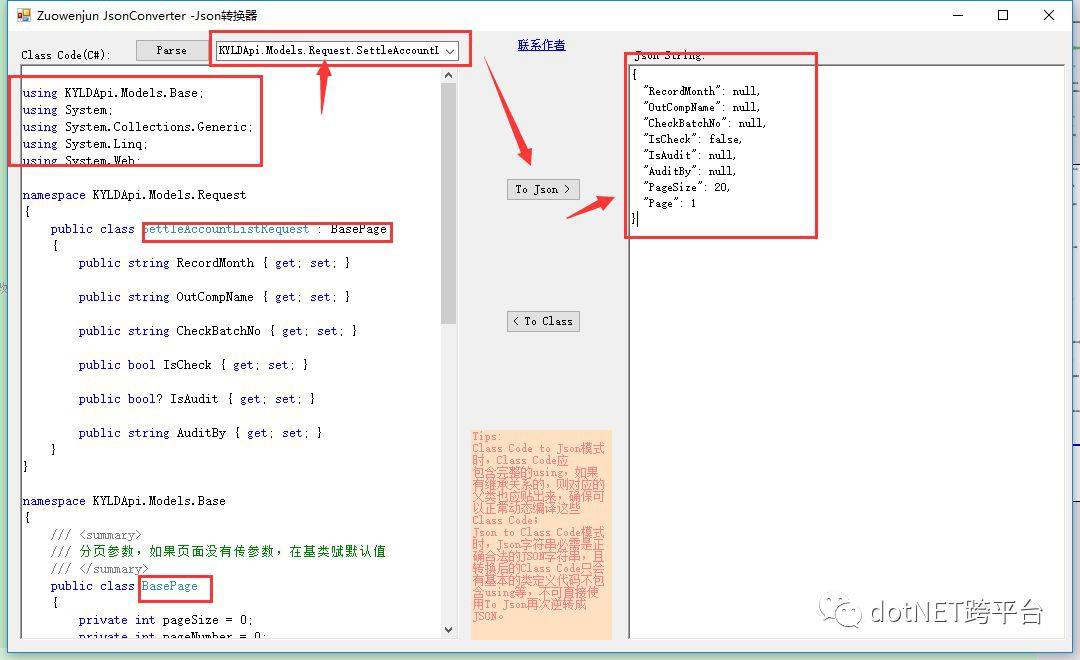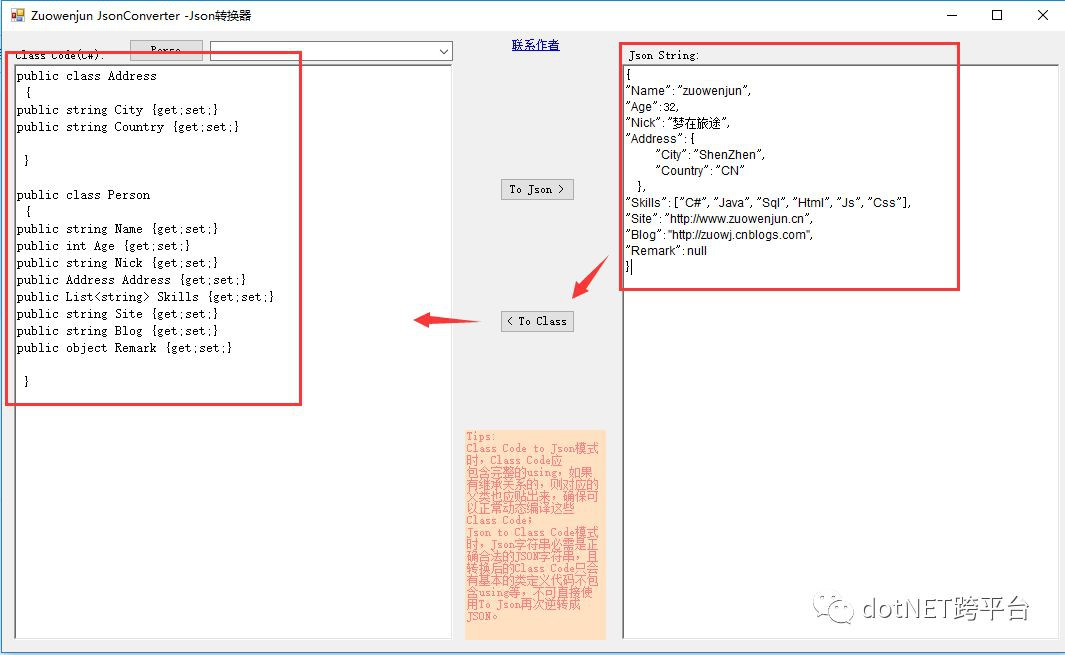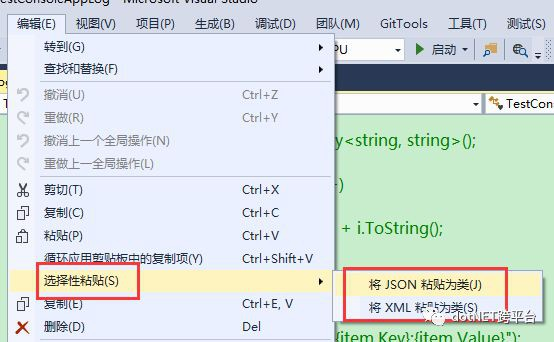# 分享基於.NET動態編譯&Newtonsoft.Json封裝實現JSON轉換器原理及JSON操作技巧

看文章標題就知道，本文的主題就是關於JSON，JSON轉換器（JsonConverter）具有將C#定義的類原始碼直接轉換成對應的JSON字串，以及將JSON字串轉換成對應的C#定義的類原始碼，而JSON操作技巧則說明如何透過JPath來快速的定位JSON的屬性節點從而達到靈活讀寫JSON目的。

## 一、JSON轉換器（JsonConverter）使用及原理介紹篇

現在都流行微服務，前後端分離，而微服務之間、前後端之間資料互動更多的是基於REST FUL風格的API，API的請求與響應一般常用格式都是JSON。當編寫了一些API後，為了能夠清楚的描述API的請求及響應資料格式（即：JSON格式），以便提供給API服務的消費者（其它微服務、前端）開發人員進行對接開發，通常是編寫API說明檔案，說明檔案中一般包含入參JSON格式說明以及響應的JSON格式說明示例，但如果API涉及數目較多，全由開發人員人工編寫，那效率就非常低下，而且不一定準確。於是就有了Swagger，在API專案中整合swagger元件，就會由swagger根據API的ACTION方法定義及註解生成標準的線上API說明檔案，具體用法請參見網上相關文章說明。當然除了swagger還有其它類似的整合式的生成線上API說明檔案，大家有興趣的話可以去網上找找資源。雖說swagger元件確實解放了開發人員的雙手，無需人工編寫就自動生成線上API檔案，但我認為還是有一些不足，或者說是不太方便的地方：一是必需整合到API專案中，與API專案本身有耦合與依賴，無法單獨作為API說明檔案專案，在有些情況下可能並不想依賴swagger，不想時刻把swagger生成API檔案暴露出來；二是目前都是生成的線上API檔案，如果API在某些網路環境下不可訪問（比如：受限），那線上的API檔案基本等同於沒用，雖說swagger也可以透過複雜的配置或改造支援匯出離線的API檔案，但總歸是有一定的學習成本。那有沒有什麼替代方案能解決swagger類似的線上API檔案的不足，又避免人工低效編寫的狀況呢？可能有，我（夢在旅途）沒瞭解過，但我為瞭解決上述問題，基於.NET動態編譯&Newtonsoft.Json;封裝實現了一個JSON轉換器（JsonConverter），採用人工編寫+JSON自動生成的方式來實現靈活、快速、離線編寫API說明檔案。class類原始碼轉換成Json字串：先將專案中定義的class類原始碼複製貼上到Class Code文字框區域【註意：若有繼承或屬性本身又是另一個類，則相關的class類定義原始碼均應一同複製，using合併，namespace允許多個，目的是確保可以動態編譯透過】，然後點選上方的【Parse】按鈕，以便執行動態編譯並解析出Class Code文字框區域中所包含的class Type，最後選擇需要生成JSON的class Type，點選中間的【To Json】按鈕，即可將選擇的class Type 序列化生成JSON字串並展示在右邊的Json String文字框中；Json字串轉換成class類定義原始碼：先將正確的JSON字串複製貼上到Json String文字框中，然後直接點選中間的【To Class】按鈕，彈出輸入要生成的class名對話方塊，輸入後點選確定就執行轉換邏輯，最終將轉換成功的class定義原始碼展示在左邊的Class Code文字框區域中；JsonConverter工具實現原理及程式碼說明：

class Code To Json 先利用.NET動態編譯程式集的方式，把class Code動態編譯成一個記憶體的臨時程式集Assembly，然後獲得該Assembly中的Class Type,最後透過反射建立一個Class Type空實體，再使用Newtonsoft.Json 序列化成JSON字串即可。

 `private` `List<``string``> Parse(``string` `csCode)` `{` `    ``var` `provider = ``new` `CSharpCodeProvider();` `    ``var` `cp = ``new` `CompilerParameters();` `    ``cp.GenerateExecutable = ``false``;` `    ``cp.GenerateInMemory = ``true``;` `    ``cp.IncludeDebugInformation = ``false``;` `    ``cp.ReferencedAssemblies.Add(``"System.dll"``);` `    ``cp.ReferencedAssemblies.Add(``"System.Data.dll"``);` `    ``cp.ReferencedAssemblies.Add(``"System.Linq.dll"``);` `    ``cp.ReferencedAssemblies.Add(``"System.ComponentModel.DataAnnotations.dll"``);` `    ``cp.ReferencedAssemblies.Add(``"Newtonsoft.Json.dll"``);` `    ``CompilerResults result = provider.CompileAssemblyFromSource(cp, csCode);` `    ``List<``string``> errList = ``new` `List<``string``>();` `    ``if` `(result.Errors.Count > 0)` `    ``{` `        ``foreach` `(CompilerError err ``in` `result.Errors)` `        ``{` `            ``errList.Add(``string``.Format(``"Line:{0},ErrorNumber:{1},ErrorText:{2}"``, err.Line, err.ErrorNumber, err.ErrorText));` `        ``}` `        ``MessageBox.Show(``"Compile error:\n"` `+ ``string``.Join(``"\n"``, errList));` `        ``return` `null``;` `    ``}` `    ``dyAssembly = result.CompiledAssembly;` `    ``return` `dyAssembly.GetTypes().Select(t => t.FullName).ToList();` `}` `private` `string` `ToJsonString(``string` `targetType)` `{` `    ``if` `(dyAssembly == ``null``)` `    ``{` `        ``MessageBox.Show(``"dyAssembly is null!"``);` `        ``return` `null``;` `    ``}` `    ``var` `type = dyAssembly.GetType(targetType);` `    ``var` `typeConstructor = type.GetConstructor(Type.EmptyTypes);` `    ``var` `obj = typeConstructor.Invoke(``null``);` `    ``return` `JsonConvert.SerializeObject(obj, Formatting.Indented, ``new` `JsonSerializerSettings { DateFormatString = ``"yyyy-MM-dd HH:mm:ss"` `});` `}`

Json to Class code 先使用JObject.Parse將json字串轉換為通用的JSON型別實體，然後直接透過獲取所有JSON屬性集合併遍歷這些屬性，透過判斷屬性節點的型別，若是子JSON型別【即：JObject】則建立物件屬性字串 同時遞迴查詢子物件，若是陣列型別【即：JArray】則建立List集合屬性字串，同時進一步判斷陣列的元素型別，若是子JSON型別【即：JObject】則仍是遞迴查詢子物件，最終拼接成所有類及其子類的class定義原始碼字串。核心程式碼如下：

 `private` `string` `ToClassCode(JObject jObject, ``string` `className)` `     ``{` `         ``var` `classCodes = ``new` `Dictionary<``string``, ``string``>();` `         ``classCodes.Add(className, BuildClassCode(jObject, className, classCodes));` `         ``StringBuilder codeBuidler = ``new` `StringBuilder();` `         ``foreach` `(``var` `code ``in` `classCodes)` `         ``{` `             ``codeBuidler.AppendLine(code.Value);` `         ``}` `         ``return` `codeBuidler.ToString();` `     ``}` `     ``private` `Dictionarystring> jTokenBaseTypeMappings = new Dictionarystring> {` `         ``{ JTokenType.Integer,``"int"` `},{ JTokenType.Date,``"DateTime"` `},{ JTokenType.Bytes,``"byte[]"``},{ JTokenType.Boolean,``"bool"``},{ JTokenType.String,``"string"``},` `         ``{ JTokenType.Null,``"object"``},{ JTokenType.Float,``"float"``},{ JTokenType.TimeSpan,``"long"``}` `     ``};` `     ``private` `string` `BuildClassCode(JObject jObject, ``string` `className, Dictionary<``string``, ``string``> classCodes)` `     ``{` `         ``StringBuilder classBuidler = ``new` `StringBuilder();` `         ``classBuidler.Append(``"public class "` `+ className + ``" \r\n { \r\n"``);` `         ``foreach` `(``var` `jProp ``in` `jObject.Properties())` `         ``{` `             ``string` `propClassName = ``"object"``;` `             ``if` `(jProp.Value.Type == JTokenType.Object)` `             ``{` `                 ``if` `(jProp.Value.HasValues)` `                 ``{` `                     ``propClassName = GetClassName(jProp.Name);` `                     ``if` `(classCodes.ContainsKey(propClassName))` `                     ``{` `                         ``propClassName = className + propClassName;` `                     ``}` `                     ``classCodes.Add(propClassName, BuildClassCode((JObject)jProp.Value, propClassName, classCodes));` `                 ``}` `                 ``classBuidler.AppendFormat(``"public {0} {1} {2}\r\n"``, propClassName, jProp.Name, ``"{get;set;}"``);` `             ``}` `             ``else` `if` `(jProp.Value.Type == JTokenType.Array)` `             ``{` `                 ``if` `(jProp.Value.HasValues)` `                 ``{` `                     ``var` `jPropArrItem = jProp.Value.First;` `                     ``if` `(jPropArrItem.Type == JTokenType.Object)` `                     ``{` `                         ``propClassName = GetClassName(jProp.Name);` `                         ``if` `(classCodes.ContainsKey(propClassName))` `                         ``{` `                             ``propClassName = className + propClassName;` `                         ``}` `                         ``propClassName += ``"Item"``;` `                         ``classCodes.Add(propClassName, BuildClassCode((JObject)jPropArrItem, propClassName, classCodes));` `                     ``}` `                     ``else` `                     ``{` `                         ``if` `(jTokenBaseTypeMappings.ContainsKey(jPropArrItem.Type))` `                         ``{` `                             ``propClassName = jTokenBaseTypeMappings[jPropArrItem.Type];` `                         ``}` `                         ``else` `                         ``{` `                             ``propClassName = jPropArrItem.Type.ToString();` `                         ``}` `                     ``}` `                 ``}` `                 ``classBuidler.AppendFormat(``"public List {1} {2}\r\n"``, propClassName, jProp.Name, ``"{get;set;}"``);` `             ``}` `             ``else` `             ``{` `                 ``if` `(jTokenBaseTypeMappings.ContainsKey(jProp.Value.Type))` `                 ``{` `                     ``propClassName = jTokenBaseTypeMappings[jProp.Value.Type];` `                 ``}` `                 ``else` `                 ``{` `                     ``propClassName = jProp.Value.Type.ToString();` `                 ``}` `                 ``classBuidler.AppendFormat(``"public {0} {1} {2} \r\n"``, propClassName, jProp.Name, ``"{get;set;}"``);` `             ``}` `         ``}` `         ``classBuidler.Append(``"\r\n } \r\n"``);` `         ``return` `classBuidler.ToString();` `     ``}`

把JSON字串轉換為class類原始碼，除了我這個工具外，網上也有一些線上的轉換網頁可以使用，另外我再分享一個小技巧，即：直接利用VS的編輯-》【選擇性貼上】，然後選擇貼上成JSON類或XML即可，選單位置：JsonConverter工具已開源並上傳至GitHub，地址：https://github.com/zuowj/JsonConverter

## 二、JSON操作技巧篇

場景一：如果已有JSON字串，現在需要獲得指定屬性節點的資料，且指定的屬性名不確定，由外部傳入或邏輯計算出來的【即：不能直接在程式碼中寫死要獲取的屬性邏輯】，那麼這時該如何快速的按需獲取任意JSON節點的資料呢？

重點來了，我們可以透過JPath運算式來快速定位查詢JSON的屬性節點，就像XML利用XPath一樣查詢DOM節點。

JPath運算式是什麼呢？ 詳見：https://goessner.net/articles/JsonPath/  ，Xpath與JSONPath對比用法如下圖示（圖片來源於https://goessner.net/articles/JsonPath/文中）：

 `using` `Newtonsoft.Json.Linq;` `using` `System;` `using` `System.Collections.Generic;` `using` `System.Linq;` `namespace` `Zuowj.EasyUtils` `{` `    ``///` `    ``/// JObject擴充套件類` `    ``/// author:zuowenjun` `    ``/// 2019-6-15` `    ``/// `

`    ``public` `static` `class` `JObjectExtensions`

`    ``{`

`        ``///`

`        ``/// 根據Jpath查詢JSON指定的屬性節點值`

`        ``/// `

`        ``///`

`        ``///`

`        ``/// `

`        ``public` `static` `IEnumerable FindJsonNodeValues(``this` `JObject jObj, ``string` `fieldPath)`

`        ``{`

`            ``var` `tks = jObj.SelectTokens(fieldPath, ``true``);`

`            ``List nodeValues = ``new` `List();`

`            ``foreach` `(``var` `tk ``in` `tks)`

`            ``{`

`                ``if` `(tk ``is` `JProperty)`

`                ``{`

`                    ``var` `jProp = tk ``as` `JProperty;`

`                    ``nodeValues.Add(jProp.Value);`

`                ``}`

`                ``else`

`                ``{`

`                    ``nodeValues.Add(tk);`

`                ``}`

`            ``}`

`            ``return` `nodeValues;`

`        ``}`

`        ``///`

`        ``/// 根據Jpath查詢JSON指定的屬性節點並賦值`

`        ``/// `

`        ``///`

`        ``///`

`        ``///`

`        ``public` `static` `void` `SetJsonNodeValue(``this` `JObject jObj, ``string` `fieldPath, JToken value)`

`        ``{`

`            ``var` `tks = jObj.SelectTokens(fieldPath, ``true``);`

`            ``JArray targetJArray = ``null``;`

`            ``List<``int``> arrIndexs = ``new` `List<``int``>();`

`            ``foreach` `(``var` `tk ``in` `tks)`

`            ``{`

`                ``JProperty jProp = ``null``;`

`                ``if` `(tk ``is` `JProperty)`

`                ``{`

`                    ``jProp = tk ``as` `JProperty;`

`                ``}`

`                ``else` `if` `(tk.Parent ``is` `JProperty)`

`                ``{`

`                    ``jProp = (tk.Parent ``as` `JProperty);`

`                ``}`

`                ``else` `if` `(tk.Parent ``is` `JObject)`

`                ``{`

`                    ``jProp = (tk.Parent ``as` `JObject).Property(tk.Path.Substring(tk.Path.LastIndexOf(``'.'``) + 1));`

`                ``}`

`                ``if` `(jProp != ``null``)`

`                ``{`

`                    ``jProp.Value = value;`

`                ``}`

`                ``else` `if` `(tk.Parent ``is` `JArray)`

`                ``{`

`                    ``targetJArray = tk.Parent ``as` `JArray;`

`                    ``arrIndexs.Add(targetJArray.IndexOf(tk));`

`                ``}`

`                ``else`

`                ``{`

`                    ``throw` `new` `Exception(``"無法識別的元素"``);`

`                ``}`

`            ``}`

`            ``if` `(targetJArray != ``null` `&& arrIndexs.Count > 0)`

`            ``{`

`                ``foreach` `(``int` `i ``in` `arrIndexs)`

`                ``{`

`                    ``targetJArray[i] = value;`

`                ``}`

`            ``}`

`        ``}`

`        ``///`

`        ``/// 在指定的JPath的屬性節點位置前或後建立新的屬性節點`

`        ``/// `

`        ``///`

`        ``///`

`        ``///`

`        ``///`

`        ``///`

`        ``/// `

`        ``public` `static` `void` `AppendJsonNode(``this` `JObject jObj, ``string` `fieldPath, ``string` `name, JToken value, ``bool` `addBefore = ``false``)`

`        ``{`

`            ``var` `nodeValues = FindJsonNodeValues(jObj, fieldPath);`

`            ``if` `(nodeValues == ``null` `|| !nodeValues.Any()) ``return``;`

`            ``foreach` `(``var` `node ``in` `nodeValues)`

`            ``{`

`                ``var` `targetNode = node;`

`                ``if` `(node ``is` `JObject)`

`                ``{`

`                    ``targetNode = node.Parent;`

`                ``}`

`                ``var` `jProp = ``new` `JProperty(name, value);`

`                ``if` `(addBefore)`

`                ``{`

`                    ``targetNode.AddBeforeSelf(jProp);`

`                ``}`

`                ``else`

`                ``{`

`                    ``targetNode.AddAfterSelf(jProp);`

`                ``}`

`            ``}`

`        ``}`

`    ``}`

`}`

控制檯輸出的結果如下：可以觀察JSON1（原JSON），JSON2（改變了JSON值）,JSON3（增加了JSON屬性節點）

 `JSON1:{` `  ``"Root"``: {` `    ``"Lv1"``: {` `      ``"col1"``: 123,` `      ``"col2"``: ``true``,` `      ``"col3"``: {` `        ``"f1"``: ``"aa"``,` `        ``"f2"``: ``"bb"``,` `        ``"f3"``: ``"cc"``,` `        ``"Lv2"``: {` `          ``"flv1"``: 1,` `          ``"flv2"``: ``"flv2-2"` `        ``}` `      ``}` `    ``}` `  ``},` `  ``"Main"``: [` `    ``{` `      ``"mf1"``: ``"x"``,` `      ``"mf2"``: ``"y"``,` `      ``"mf3"``: 123` `    ``},` `    ``{` `      ``"mf1"``: ``"x2"``,` `      ``"mf2"``: ``"y2"``,` `      ``"mf3"``: 225` `    ``}` `  ``]` `}` ```FindJsonNodeValues:1,flv2-2 ``` `JSON2:{` `  ``"Root"``: {` `    ``"Lv1"``: {` `      ``"col1"``: 123,` `      ``"col2"``: ``true``,` `      ``"col3"``: {` `        ``"f1"``: ``"aa"``,` `        ``"f2"``: ``"bb"``,` `        ``"f3"``: ``"cc"``,` `        ``"Lv2"``: {` `          ``"flv1"``: 1,` `          ``"flv2"``: ``"flv2-2"` `        ``}` `      ``}` `    ``}` `  ``},` `  ``"Main"``: [` `    ``{` `      ``"mf1"``: ``"x"``,` `      ``"mf2"``: ``"*changed value*"``,` `      ``"mf3"``: 123` `    ``},` `    ``{` `      ``"mf1"``: ``"x2"``,` `      ``"mf2"``: ``"*changed value*"``,` `      ``"mf3"``: 225` `    ``}` `  ``]` ```} ``` `JSON3:{` `  ``"Root"``: {` `    ``"Lv1"``: {` `      ``"col1"``: 123,` `      ``"col2"``: ``true``,` `      ``"col3"``: {` `        ``"f1"``: ``"aa"``,` `        ``"f2"``: ``"bb"``,` `        ``"f3"``: ``"cc"``,` `        ``"Lv2"``: {` `          ``"flv1"``: 1,` `          ``"flv2"``: ``"flv2-2"` `        ``},` `        ``"LV2-New"``: {` `          ``"flv21"``: ``"a2"``,` `          ``"flv22"``: 221,` `          ``"flv23"``: ``true` `        ``}` `      ``}` `    ``}` `  ``},` `  ``"Main"``: [` `    ``{` `      ``"mf1"``: ``"x"``,` `      ``"mf2"``: ``"*changed value*"``,` `      ``"mf3"``: 123` `    ``},` `    ``{` `      ``"mf1"``: ``"x2"``,` `      ``"mf2"``: ``"*changed value*"``,` `      ``"mf3"``: 225` `    ``}` `  ``]` `}`

好了，本文的內容就分享到這裡。